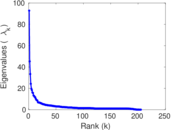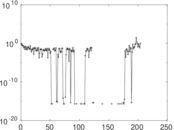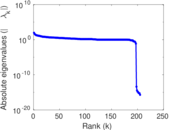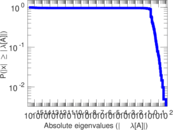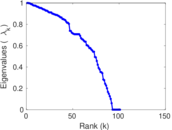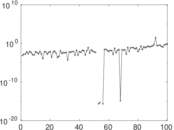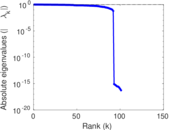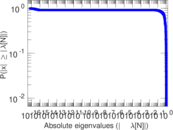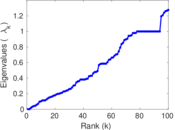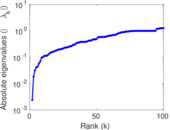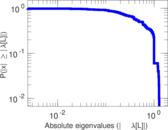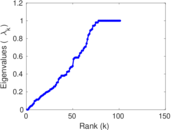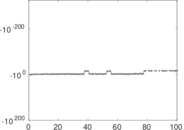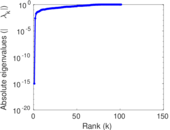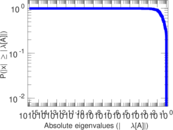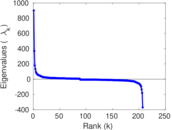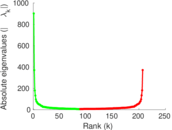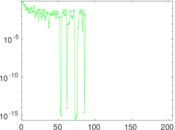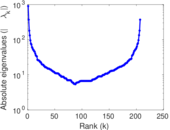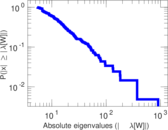# Wikibooks edits (cv)

This is the bipartite edit network of the Chuvash Wikibooks. It contains users and pages from the Chuvash Wikibooks, connected by edit events. Each edge represents an edit. The dataset includes the timestamp of each edit.

 Code `bcv` Internal name `edit-cvwikibooks` Name Wikibooks edits (cv) Data source http://dumps.wikimedia.org/ AvailabilityDataset is available for download Consistency checkDataset passed all tests Category Authorship network Dataset timestamp 2017-10-20 Node meaning User, article Edge meaning Edit Network formatBipartite, undirected Edge typeUnweighted, multiple edges Temporal dataEdges are annotated with timestamps

## Statistics

 Size n = 914 Left size n1 = 207 Right size n2 = 707 Volume m = 1,498 Unique edge count m̿ = 851 Wedge count s = 21,196 Claw count z = 1,047,161 Cross count x = 45,036,622 Square count q = 267 4-Tour count T4 = 88,694 Maximum degree dmax = 202 Maximum left degree d1max = 202 Maximum right degree d2max = 125 Average degree d = 3.277 90 Average left degree d1 = 7.236 71 Average right degree d2 = 2.118 81 Fill p = 0.005 814 87 Average edge multiplicity m̃ = 1.760 28 Size of LCC N = 580 Diameter δ = 19 50-Percentile effective diameter δ0.5 = 7.031 13 90-Percentile effective diameter δ0.9 = 10.308 6 Median distance δM = 8 Mean distance δm = 6.972 73 Gini coefficient G = 0.651 556 Balanced inequality ratio P = 0.243 658 Left balanced inequality ratio P1 = 0.214 953 Right balanced inequality ratio P2 = 0.309 079 Relative edge distribution entropy Her = 0.880 817 Power law exponent γ = 4.699 05 Tail power law exponent γt = 2.551 00 Tail power law exponent with p γ3 = 2.551 00 p-value p = 0.024 000 0 Left tail power law exponent with p γ3,1 = 2.171 00 Left p-value p1 = 0.920 000 Right tail power law exponent with p γ3,2 = 3.151 00 Right p-value p2 = 0.003 000 00 Degree assortativity ρ = −0.176 724 Degree assortativity p-value pρ = 2.117 94 × 10−7 Spectral norm α = 92.815 0 Algebraic connectivity a = 0.002 392 25 Spectral separation |λ1[A] / λ2[A]| = 2.052 45 Controllability C = 489 Relative controllability Cr = 0.553 794

## Plots

### Fruchterman–Reingold graph drawing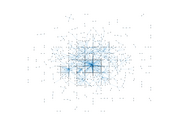### Degree distribution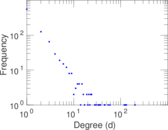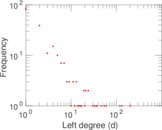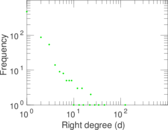### Cumulative degree distribution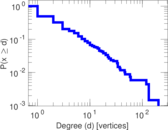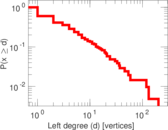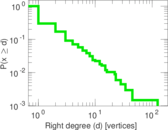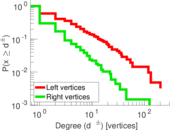### Lorenz curve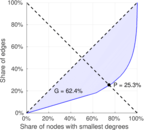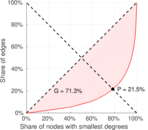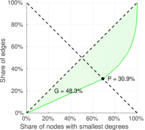### Spectral distribution of the adjacency matrix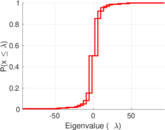### Spectral distribution of the normalized adjacency matrix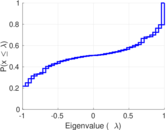### Spectral distribution of the Laplacian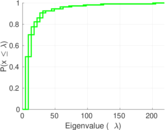### Spectral graph drawing based on the adjacency matrix### Spectral graph drawing based on the Laplacian### Spectral graph drawing based on the normalized adjacency matrix### Degree assortativity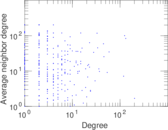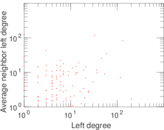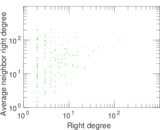### Zipf plot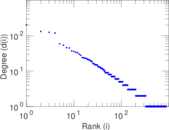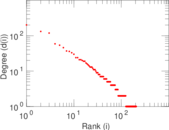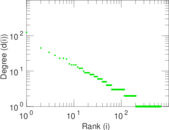### Hop distribution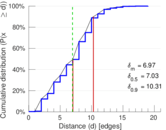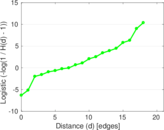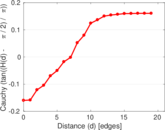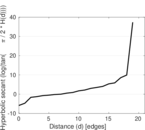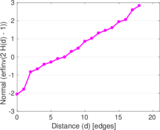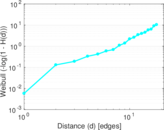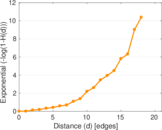### Double Laplacian graph drawing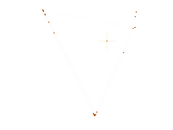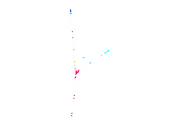### Delaunay graph drawing### Edge weight/multiplicity distribution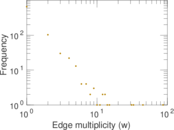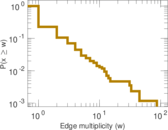### Temporal distribution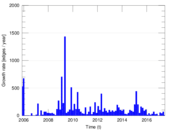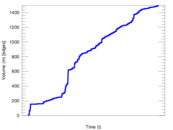### Temporal hop distribution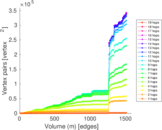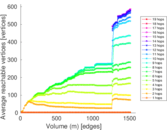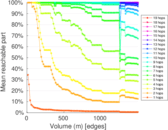### Diameter/density evolution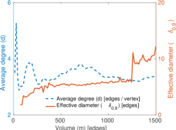### Matrix decompositions plots